Next: Collapse of the Wave Up: Wave-Particle Duality Previous: Heisenberg's Uncertainty Principle

# Schrödinger's Equation

We have seen that the wavefunction of a free particle of masssatisfies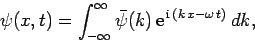(128)

where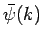is determined by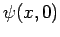, and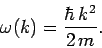(129)

Now, it follows from Eq. (128) that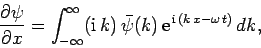(130)

and(131)

whereas(132)

Thus,(133)

where use has been made of the dispersion relation (129). Multiplying through by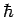, we obtain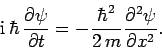(134)

This expression is known as Schrödinger's equation, since it was first introduced by Erwin Schrödinger in 1925. Schrödinger's equation is a linear, second-order, partial differential equation which governs the time evolution of a particle wavefunction, and is generally easier to solve than the integral equation (128).

Of course, Eq. (134) is only applicable to freely moving particles. Fortunately, it is fairly easy to guess the generalization of this equation for particles moving in some potential. It is plausible, from Eq. (130), that we can identifywith the differential operator. Hence, the differential operator on the right-hand side of Eq. (134) is equivalent to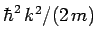. But,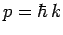. Thus, the operator is also equivalent to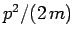, which is just the energy of a freely moving particle. However, in the presence of a potential, the particle's energy is written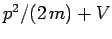. Thus, it seems reasonable to make the substitution(135)

This leads to the general form of Schrödinger's equation: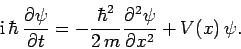(136)Next: Collapse of the Wave Up: Wave-Particle Duality Previous: Heisenberg's Uncertainty Principle
Richard Fitzpatrick 2010-07-20